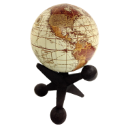# Create extent to fit all points keeping centre fixed

747
5
02-08-2019 08:01 AMNew Contributor II

Hi,

I'm trying to derive an extent out of a list of point geometries, so that all the geometries fit into the extent, keeping fixed to a provided centre. The problem I'm having with extent.union() is it, doesn't keep the extent centre fixed. And doing extent.centerAt() post all the union()s does not fit all the points. And extent.expand() does not take a point. Is there a similar solution as google.maps.LatLngBounds.extend(Point) in ARCGis to extend the extent to a given point keeping the centre fixed.

``````fitBounds: function (mapsterGeoJson, boundCen) {
var me = this;
var tempExtnt = null;
var resExtnt = this.createPointExtent(boundCen.getValue());
mapsterGeoJson.getVendorGeoJSON().graphics.forEach(function (graphics) {
tempExtnt = me.createPointExtent(graphics.geometry);
if (!resExtnt.contains(tempExtnt)) {
resExtnt.union(tempExtnt);
}
});
resExtnt.centerAt(boundCen.getValue());
me.getVendorView().whenLayerView(mapsterGeoJson.getVendorGeoJSON()).then(
function () {
me.getVendorView().goTo(resExtnt);
});
return this;
},
createPointExtent: function (pointGeometry) {
return  new this.mapObj.apiInterface.geometry.Extent({
xmin: pointGeometry.longitude,
xmax: pointGeometry.longitude,
ymin: pointGeometry.latitude,
ymax: pointGeometry.latitude
});
},‍‍‍‍‍‍‍‍‍‍‍‍‍‍‍‍‍‍‍‍‍‍‍‍‍‍‍‍‍‍‍‍‍‍‍‍‍‍‍‍‍‍‍‍‍‍‍‍‍‍‍‍‍‍‍‍‍‍‍‍‍‍‍‍‍‍‍‍‍‍‍‍‍‍‍‍‍‍‍‍‍‍‍‍‍‍‍‍‍‍‍‍‍‍‍‍‍‍‍‍‍‍‍‍‍‍‍‍‍‍‍‍‍‍‍‍‍‍‍‍‍‍‍‍‍‍‍‍‍‍‍‍‍‍‍‍‍‍‍‍‍``````
Tags (5)
1 Solution

Accepted SolutionsbyMVP Esteemed Contributor

Rupam,

I convert a point into an extent using a default 6 pixel tolerance. Normally I use this for getting an extent from a mouse click, but I think it will help in your situation.

``````pointToExtent: function (pointGeometry, distance) {
var clickOffset = distance || 6;
var centerPoint = pointGeometry.clone();
var mapWidth = me.getVendorView().extent.width;
var pixelWidth = mapWidth / me.getVendorView().width;
var tolerance = clickOffset * pixelWidth;
var queryExtent = new this.mapObj.apiInterface.geometry.Extent({xmin: 1, ymin: 1, xmax: tolerance, ymax: tolerance, spatialReference: pointGeometry.spatialReference});
return queryExtent.centerAt(centerPoint);
},‍‍‍‍‍‍‍‍‍‍‍‍‍‍‍‍‍‍``````
5 RepliesbyMVP Esteemed Contributor

Rupam,

The one way I can think of is to clone the resExtnt to a new var before you center it. then union the centered resExtnt and the cloned one.New Contributor II

Robert,

Tried it, but doesn't work.

``````setBounds: function (mapsterGeoJson, boundCen) {
var me = this;
var tempExtnt = null;
var resExtnt = this.createPointExtent(boundCen.getValue());
mapsterGeoJson.getVendorGeoJSON().graphics.forEach(function (graphics) {
tempExtnt = me.createPointExtent(graphics.geometry);
if (!resExtnt.contains(tempExtnt)) {
resExtnt.union(tempExtnt);
}
});
var clonedExtent = resExtnt.clone();
resExtnt.centerAt(boundCen.getValue());
resExtnt.union(clonedExtent);
me.getVendorView().whenLayerView(mapsterGeoJson.getVendorGeoJSON()).then(
function () {
me.getVendorView().goTo(resExtnt);
});
return this;
},‍‍‍‍‍‍‍‍‍‍‍‍‍‍‍‍‍‍‍``````

Also couldn't use extent.expand() as it requires a factor to multiply and I have to test all the points after expand till all the points fit. And also the point extents have zero height and width so expand will not modify the extent at all.byMVP Esteemed Contributor

Rupam,

I convert a point into an extent using a default 6 pixel tolerance. Normally I use this for getting an extent from a mouse click, but I think it will help in your situation.

``````pointToExtent: function (pointGeometry, distance) {
var clickOffset = distance || 6;
var centerPoint = pointGeometry.clone();
var mapWidth = me.getVendorView().extent.width;
var pixelWidth = mapWidth / me.getVendorView().width;
var tolerance = clickOffset * pixelWidth;
var queryExtent = new this.mapObj.apiInterface.geometry.Extent({xmin: 1, ymin: 1, xmax: tolerance, ymax: tolerance, spatialReference: pointGeometry.spatialReference});
return queryExtent.centerAt(centerPoint);
},‍‍‍‍‍‍‍‍‍‍‍‍‍‍‍‍‍‍``````New Contributor II

Hi Robert,

The solution worked. So the code now looks as follows.

``````setBounds: function (mapsterGeoJson, singularity) {
var me = this;
me.getVendorView().whenLayerView(mapsterGeoJson.getVendorGeoJSON()).then(function () {
var blackhole = me.createPointExtent(singularity.getValue()); //Create extent at the center
var maxDist = 0, tempDist = 0;
mapsterGeoJson.getVendorGeoJSON().graphics.forEach(function (graphics) {
tempDist = me.mapObj.apiInterface.geometry.geometryEngine.distance(singularity.getValue(),
graphics.geometry);
if (tempDist > maxDist) {
maxDist = tempDist;
}
}); //Find the farthest distance from center
/*Expand the extent to the farthest geometry. And acounting for device aspect ratio.*/
blackhole.expand((maxDist + (maxDist * 1.5)) / blackhole.width);
me.getVendorView().goTo(blackhole);
});
return this;
},
createPointExtent: function (pointGeometry) {
var clickOffset = 6;
var centerPoint = pointGeometry.clone();
var mapWidth = this.getVendorView().extent.width;
var pixelWidth = mapWidth / this.getVendorView().width;
var tolerance = clickOffset * pixelWidth;
var queryExtent = new this.mapObj.apiInterface.geometry.Extent({
xmin: 1,
ymin: 1,
xmax: tolerance,
ymax: tolerance,
spatialReference: pointGeometry.spatialReference
});
return queryExtent.centerAt(centerPoint);
},‍‍‍‍‍‍‍‍‍‍‍‍‍‍‍‍‍‍‍‍‍‍‍‍‍‍‍‍‍‍‍‍‍``````

Thank you for the help.byMVP Esteemed Contributor